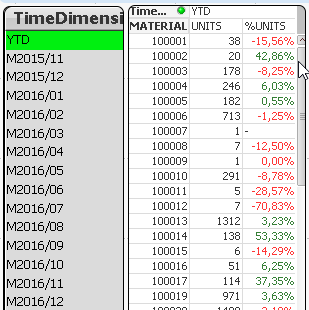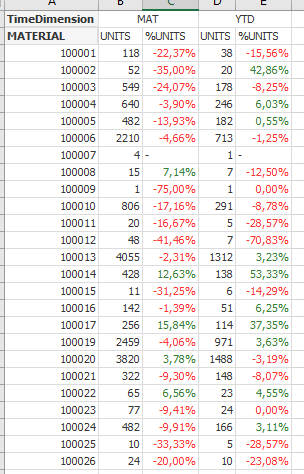# QlikView App Dev

Discussion Board for collaboration related to QlikView App Development.

Announcements
Join us for a live Q&A! September 21, 10 AM ET - Onboarding Fast in Qlik Sense SaaS! REGISTER
cancel
Showing results for
Did you mean:Contributor II

## How to calculate % change between 2 timeperiods when only one period is selected and shown as dimension

Hi Community,

i am struggling with a requirement looking simple on first sight but turned into some hairy problem for me.

In my datamodel theres a FACT table with basic information like Date, Material and Units.

I also use a TIME (Calendar) table with a few calculated periods for easier calculation and filtering. So far so good.

Because a few objects should be very generic concering the usage of TimeDimensions and the comparison of them, I linked a Table "ZeitDim" with the TIME table where the user can use the field "Timedimension" to choose the periods very generic.

For absolute values this is fine, but now I want to calculate the  relative change in % between the choosen Timeperiod and the Timeperiod defined as the correct related Timeperiod in the past.

E.g. if the user selects Timedimension='YTD', the values where Timedimension='YTD' should be divided by the values with Timedimension='YTD VJ'.

As a reference I prepared a taple (the suffix VP means previous period):But only one or more of the Timedimensions (one or all 4) should be filtered from the user.

The wanted solution should look like that:YTD VJ is not selected.

The not wanted standard looks more or less like that:I tested and tried a lot with set analysis and alternate state but without luck and success.

It seems that QlikView always expects the selected values in a dimension for calculation.

Hope my requirement is clear decribed and one of you maybe has better ideas as I had.

Rainer

1 Solution

Accepted SolutionsMVP

Try this

(Sum([round(UNITS /22)])/After(Sum({<TimeDimension = p(TimeDimension)+p(TimeDimensionVP)>}[round(UNITS /22)]))-1)

16 RepliesMVP

Try this

(Sum([round(UNITS /22)])/After(Sum({<TimeDimension = p(TimeDimension)+p(TimeDimensionVP)>}[round(UNITS /22)]))-1)Contributor II
Author

Hi Sunny,

many thanks for the extreme quick reply. I've tried your solution and it looks like this is exactly what I need.

That helps a lot!

Best regards,

RainerSpecialist II

One more way other than this

( sum({<TimeDimension={\$(=only(TimeDimension))}>}[round(UNITS /22)])/

sum({<TimeDimension={'\$(=maxstring(TimeDimensionVP))'}>} total<MATERIAL> [round(UNITS /22)]) - avg({<TimeDimension={\$(=only(TimeDimension))}>}1) )Contributor II
Author

Sorry for my late reply and many thanks for your support and time!

Both solutions are working fine when only one TimeDimension ist selected.
But if I would like to see 2 different Periods like YTD and MAT the result is like that:Additionally if there are UNITS in the selected Timedimension but not in the previous one, the %value is.

BR,

RainerMVP

What would you like to see when 2 different periods are selected? Will you be able to elaborate?Contributor II
Author

As an example I would like to select YTD and MAT (and maybe more values)  as Timeperiod and the result should be:

1 column YTD (%difference Timedimension = "YTD" and the related Timedimension before which is "YTD VP")

and

a second column MAT (%difference Timedimension = "MAT" and the related Timedimension before which is "MAT VP").

The solution should be so flexible that a selection of more Timendimensions will work, also with more complex forulas, e.g. for Marketshare etc.

So the result in this case for selected values YTD and MAT should look like this:Hope that makes it much clearer what I woul like to have.

BR and many thanks,
RainerMVP

Does this solve the issue?

(sum([round(UNITS /22)])/after(sum({<TimeDimension = p(TimeDimension)+p(TimeDimensionVP)>}[round(UNITS /22)]))-1) * Avg(1)Contributor II
Author

I've tested it and it worked like expected. Many thanks -  that is really helpful!

Maybe you could give me (hopefully) just 1 last advise:

I would like to use this solution also für a formula like this which calcuates an share/ratio from a selected product compared to all products:

sum(UNITS ) / sum({<PRODUKT=>} TOTAL UNITS)

How could that formula fit into the formula we have now

(sum([round(UNITS /22)])/after(sum({<TimeDimension = p(TimeDimension)+p(TimeDimensionVP)>}[round(UNITS /22)]))-1) * Avg(1)MVP

Not entirely sure, but have you tried this?

((Sum(UNITS)/Sum({<PRODUKT=>} TOTAL UNITS))/

After(Sum({<TimeDimension = p(TimeDimension)+p(TimeDimensionVP)>}UNITS)/Sum({<PRODUKT, TimeDimension = p(TimeDimension)+p(TimeDimensionVP)>} TOTAL UNITS)) -1) * Avg(1)Community Browser